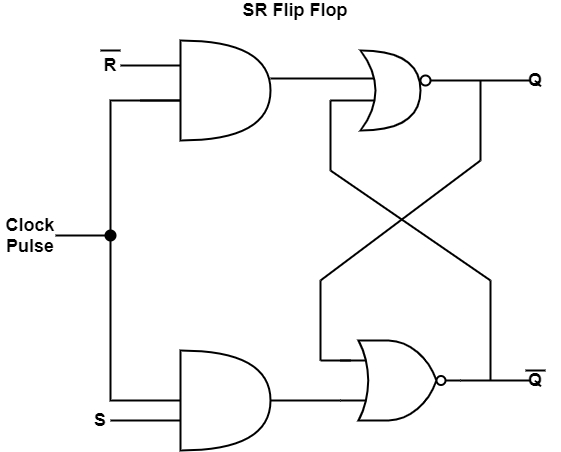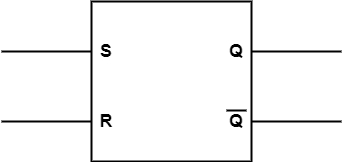# What is Set-Reset (SR) Flip-flop?

Flip flops are an application of logic gates. A flip-flop circuit can stay in a binary state continually (as long as power is transferred to the circuit) before conducted by an input signal to switch states. S-R flip-flop represents SET-RESET flip-flops. The SET-RESET flip-flop includes two NOR gates and also two NAND gates. These flip-flops are also known as S-R Latch.

The SR flip-flop has two inputs such as the ‘Set’ input and a ‘Reset’ input. The two outputs of SR flip-flop are the main output Q and its complement $\overline{Q}$.

The diagram shows the circuit diagram of an SR flip-flop.The truth table of SR flip flop is shown in the table.

SRQN.1$\overline{Q_{N\cdot1}}$
00QN$\overline{Q_{N}}$
0101
1010
11IndeterminateIndeterminate

A team of cross-coupled NOR gates can describe an SR flip-flop, wherein, the output of one gate is related to one of the two inputs of the other gate and vice versa. The complementary input of one NOR gate is ‘R’ while the complementary input of the other gate is ‘S’.

The input ‘R’ makes the output Q and the gate with the ‘S’ input makes the output $\overline{Q}$.

The logic symbol of the SR flip-flop is shown in the figure.